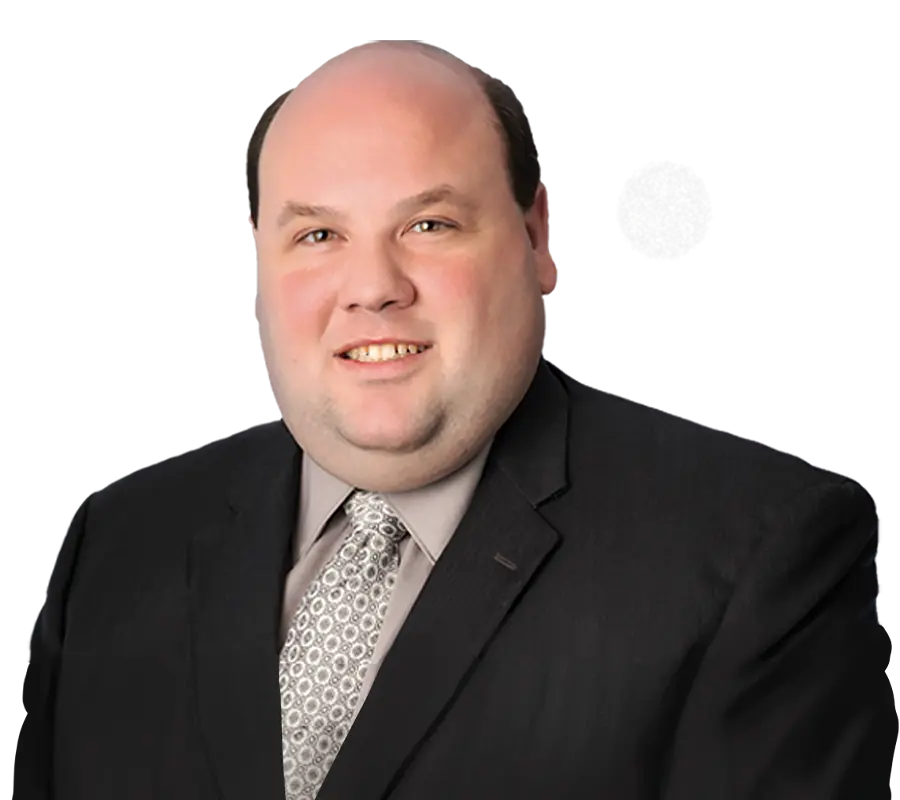# HRL Laboratories Makes a Major Breakthrough in Practical Quantum ComputingZuber Lawler

## A major breakthrough in quantum computing hardware was achieved this year when HRL Laboratories demonstrated universal control of encoded spin qubits.

To explain what this breakthrough means, it’s helpful to review how classical computers work, and the different ways in which quantum computers work. A classical computer uses bits of information coded as 1 or 0, and logical gates that take one or two binary inputs and generate a result, such as an AND gate that outputs 1 if both inputs are 1, and outputs 0 if either input is 0. In a typical classical computer, bits are physically represented by electrical voltage, and logic gates are physically created using electrical circuits that output the desired electrical voltage as a function of the input voltages. Computer scientists determined early on that any logical operation can be expressed as a combination of AND and NOT gates, and so electrical implementation of those two gates was sufficient to perform any classical computing operation.

A quantum computer in contrast, uses “qubits” that exist in a superposition of a “1” and a “0” state, and quantum logic gates that generate an output qubit based on a function of one or more input qubits.

## A significant challenge in moving from quantum computing theory to practice has been implementing physical qubit and physical quantum logic gates, as the nature of quantum effects requires information to be stored by subatomic particles kept isolated from any external force that would alter the value of the qubit.

A paper published in 1995, “Elementary gates for quantum computation” by Barenco et al, demonstrated that any quantum operation could be expressed a combination of all one-bit quantum gates and the controlled-not gate, and so physical implementation of those two quantum gates would be sufficient to implement a physical quantum computer capable of performing any quantum computation.

HRL’s research, published in Nature under the title “Universal logic with encoded spin qubits in silicon,” demonstrated a new method of qubit implementation and control using qubits consisting of a triplet of quantum dots, each quantum dot containing a single electron with a controlled spin. By using precisely calibrated voltage pulses to modify the quantum dots’ spins, HRL’s researchers were able to demonstrate an implementation of the two basic quantum logic gates with sufficient speed, error tolerance, uniformity, and scalability to demonstrate a major advance towards an effective commercial quantum computer.

HRL’s invention appears to be a significant advance in moving from quantum computing theory to practice. HRL’s group leader Thaddeus Ladd was quoted as saying that he believes this new qubit technology to be the best-balanced approach towards a practical quantum computer: “Real challenges remain in improving error, scale, speed, uniformity, crosstalk, and other aspects, but none of these requires a miracle. For many other kinds of qubits, there is at least one aspect that still looks really, really hard.” We look forward to continued competition between different qubit and quantum logic implementations moving the field forward from vision to practical reality.

Do you have an article you’d like to submit to DCLC for consideration?

If so, please submit one here!